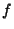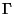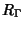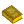## Modular Function

A function is said to be modular (or elliptic modular'') if it satisfies:

1.is Meromorphic in the upper half-Plane,

2.for every Matrix A in the modular group,
3. The Laurent Series ofhas the form(Apostol 1997, p. 34). Every Rational Function of Klein's Absolute Invariantis a modular function, and every modular function can be expressed as a Rational Function of(Apostol 1997, p. 40).

An important property of modular functions is that ifis modular and not identically 0, then the number of zeros ofis equal to the number of poles ofin the Closure of the fundamental region(Apostol 1997, p. 34).

ReferencesModular Functions

Apostol, T. M. Modular Functions and Dirichlet Series in Number Theory. New York: Springer-Verlag, 1976.

Borwein, J. M. and Borwein, P. B. Pi and the AGM: A Study in Analytic Number Theory and Computational Complexity. New York: Wiley, 1987.

Rankin, R. A. Modular Forms and Functions. Cambridge, England: Cambridge University Press, 1977.

Schoeneberg, B. Elliptic Modular Functions: An Introduction. Berlin: New York: Springer-Verlag, 1974.